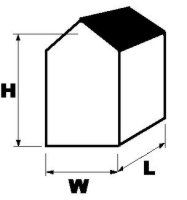01224 749051

FINANCE AVAILABLE (£1000 minimum)

# Heater Size Calculation

## (BTU CALCULATOR)To calculate the heater size required for your building, use the following formula :-

V x dT x K x B = Heater Size in BTU

• V = Volume in cubic metres (width x length x height of building in metres)
(or calculate in cubic feet then divide by 35)
use the average roof height between eaves and roof apex

• dT = difference between outdoor and required indoor temperature in degrees C
normally use a figure of 20. i.e. heating from minus five degrees outdoors to plus fifteen degrees in workshop

• K = coefficient determined by the building construction
- K = 3.5 for simple non-insulated building in wood or corrugated metal
- K = 2.5 for poorly insulated building, single brick layer, simple windows, simple roof
- K = 1.5 for moderately insulated building, double brick layer, few windows, closed roof
- K = 0.8 for well insulated , advanced construction building
select the value that best describes your building

• B = 4 (multiplication factor to arrive at BTUs)
always multiply by this figure

• Then divide your answer in BTUs by 3412 to convert to KW

Approximate conversion between imperial and metric units
10,000 BTU = 3KW
10KW = 34,000 BTU